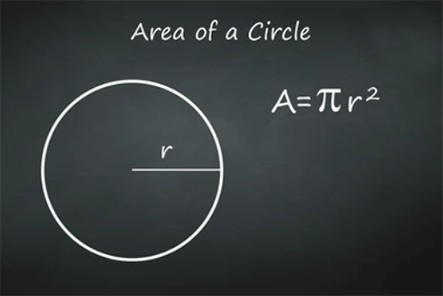HomeCareerWays to Calculate the Circumference of a Circle

# Ways to Calculate the Circumference of a Circle

A shape that consists of all the points in a plane that are at a specific distance from a given point is called a circle. Alternatively, it can be defined as the curve that is traced by a point in such a way that the distance from a given point is always constant. In this article, we will not only see how to calculate the circumference of a circle but also go through topics associated with the same.

Related Post: 10 Best Education Apps

## Circumference of a Circle

The circumference of a circle can be described as the distance around it. When we measure the circumference of a circle, we are basically trying to find the length of the boundary of the curved geometrical shape. The circumference is analogous to a polygon’s perimeter as the principle of measuring both remains the same.

The formula is given as: Circumference of a circle = 2(pi)(r) = (pi)d

where r is the radius of the circle, d is the diameter, and the value of pi is 3.14.

The radius of a circle is a line segment that joins the center of the circle to any point on the circle’s circumference. It is half of the length of the diameter. The diameter of a circle is a line segment, the endpoints of which lie on the circle itself, and it passes through the center. It is the longest chord of the circle and also represents the largest distance between two points on the circle. It is double the length of the radius.

## Area of a CircleThe area of circle can be defined as the space that is covered by the circle. In other words, it is the region that is enclosed within the boundary of a circle. The formula for the area of a circle is given by

Area of a circle = (pi)r2

Also Read: Avishkaar: India’s First Robotics Educational Institute for Kids

## The perimeter of a Semi-Circle

When we divide a circle into two equal parts, we get a semi-circle. Hence, the perimeter or circumference of a semi-circle will be half of the perimeter of a circle.

Perimeter of a semi-circle = (pi)(r) =½ (pi)(d)

## Area of a Semicircle

The area of a semi-circle is defined as the region enclosed by a semi-circle in 2D space. The formula is given by

Area of semicircle = ½ (pi)(r2)

## Methods to Study

Learning about circles can prove to be very confusing; however, if given the right guidance, a student will master this topic in no time. The best way to combat this problem of confusion is to avail the services of a reliable tuition class such as Cuemath. Cuemath is an amazing online educational platform that provides quality education to students at the best price. The certified tutors use several resources such as interactive worksheets, online puzzles, apps, and visual simulations to deliver an impactful lecture. Students get the chance to work at their own pace and maintain a flexible schedule so that they do not get pressures. They are also given the opportunity to experience several different types of practice questions that help them build strong foundations for the topic.

#### Conclusion

Circles can prove to be one of the most exciting topics to explore. By learning about circles, you start developing your foundation for Geometry. Hence, it is imperative for young minds to have crystal clear concepts on the same. Once a student is confident in solving simple problems, he will be able to use manipulations for solving complicated problems. Hopefully, this article gives you an idea of approaching the topic, and I wish you all the best!

16,985Fans
1,850Followers
2,458Followers